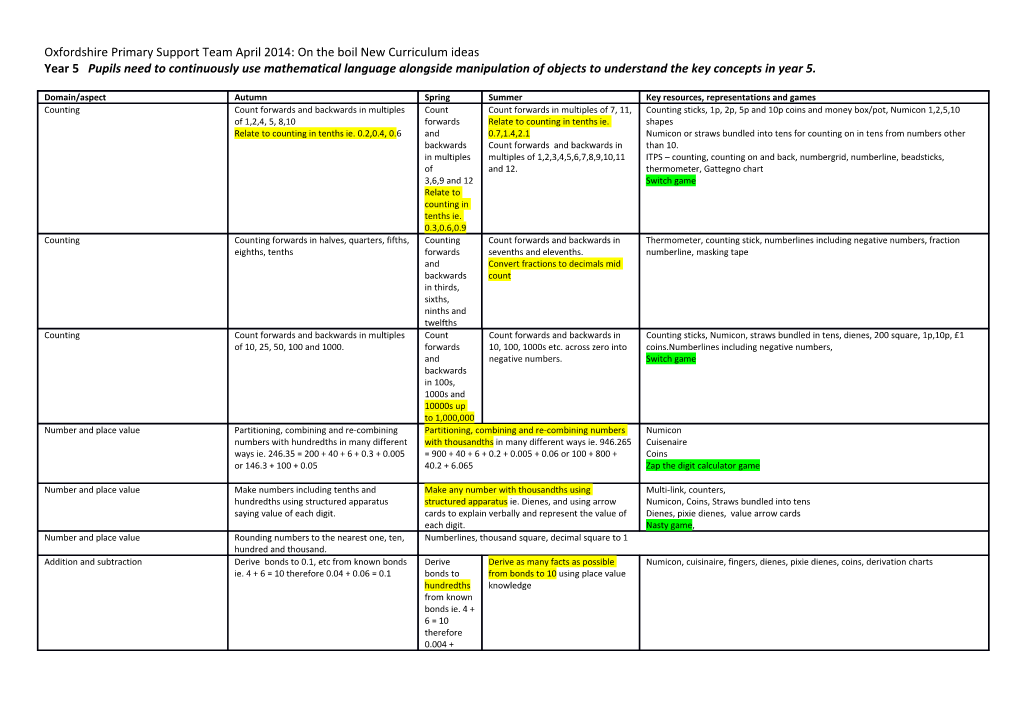# Oxfordshire Primary Support Team April 2014: on the Boil New Curriculum IdeasOxfordshire Primary Support Team April 2014: On the boil New Curriculum ideas

Year 5 Pupils need to continuously use mathematical language alongside manipulation of objects to understand the key concepts in year 5.

Domain/aspect / Autumn / Spring / Summer / Key resources, representations and games
Counting / Count forwards and backwards in multiples of 1,2,4, 5, 8,10
Relate to counting in tenths ie. 0.2,0.4, 0.6 / Count forwards and backwards in multiples of
3,6,9 and 12
Relate to counting in tenths ie. 0.3,0.6,0.9 / Count forwards in multiples of 7, 11,
Relate to counting in tenths ie. 0.7,1.4,2.1
Count forwards and backwards in multiples of 1,2,3,4,5,6,7,8,9,10,11 and 12. / Counting sticks, 1p, 2p, 5p and 10p coins and money box/pot, Numicon 1,2,5,10 shapes
Numicon or straws bundled into tens for counting on in tens from numbers other than 10.
ITPS – counting, counting on and back, numbergrid, numberline, beadsticks, thermometer, Gattegno chart
Switch game
Counting / Counting forwards in halves, quarters, fifths, eighths, tenths / Counting forwards and backwards in thirds, sixths, ninths and twelfths / Count forwards and backwards in sevenths and elevenths.
Convert fractions to decimals mid count / Thermometer, counting stick, numberlines including negative numbers, fraction numberline, masking tape
Counting / Count forwards and backwards in multiples of 10, 25, 50, 100 and 1000. / Count forwards and backwards in 100s, 1000s and 10000s up to 1,000,000 / Count forwards and backwards in 10, 100, 1000s etc. across zero into negative numbers. / Counting sticks, Numicon, straws bundled in tens, dienes, 200 square, 1p,10p, £1 coins.Numberlines including negative numbers,
Switch game
Number and place value / Partitioning, combining and re-combining numbers with hundredths in many different ways ie. 246.35 = 200 + 40 + 6 + 0.3 + 0.005 or 146.3 + 100 + 0.05 / Partitioning, combining and re-combining numbers with thousandths in many different ways ie. 946.265 = 900 + 40 + 6 + 0.2 + 0.005 + 0.06 or 100 + 800 + 40.2 + 6.065 / Numicon
Cuisenaire
Coins
Zap the digit calculator game
Number and place value / Make numbers including tenths and hundredths using structured apparatus saying value of each digit. / Make any number with thousandths using structured apparatus ie. Dienes, and using arrow cards to explain verbally and represent the value of each digit. / Multi-link, counters,
Numicon, Coins, Straws bundled into tens
Dienes, pixie dienes, value arrow cards
Nasty game,
Number and place value / Rounding numbers to the nearest one, ten, hundred and thousand. / Numberlines, thousand square, decimal square to 1
Addition and subtraction / Derive bonds to 0.1, etc from known bonds ie. 4 + 6 = 10 therefore 0.04 + 0.06 = 0.1 / Derive bonds to hundredths from known bonds ie. 4 + 6 = 10 therefore 0.004 + 0.006 = 0.01 / Derive as many facts as possible from bonds to 10 using place value knowledge / Numicon, cuisinaire, fingers, dienes, pixie dienes, coins, derivation charts
Addition and subtraction / Children practice selecting which mental calculation strategy is the most efficient when presented with increasingly challenging calculations ie. Round and adjust, find the difference, reorder, partition, count on, count back, doubles, near doubles, halves and bonds. / Bead strings, Numicon, Cuisenaire, number lines, dienes,
Teaching children to calculate mentally 2010 P35-38
Multiplication and division / Use key vocabulary – sum, product, difference – to practice finding the sum, product difference of two single digit numbers. / Use key vocabulary – sum, product, difference, square, cube to practice finding the sum, product, difference, square and cube of given numbers. / Dienes, pixie dienes, Cuisenaire, coins, place value charts, arrow cards
Multiplication and division / Rapid recall of all multiplication facts up to 12 x 12 / Rapid recall of known factors within childrens’ multiplication facts. / Identify prime numbers within a given range and square numbers up to 144. / 2p and 10p coins, Numicon, fingers, money pots
Multiplication squares, times table charts, arrays, numberlines
Measurement / Convert hours to minutes, minutes to seconds etc., / Conversion within mass ie. How many mls in a litre / Conversion within lengths ie. 1km = how many m? / Measurement equipment, scales,
ITP - measurement